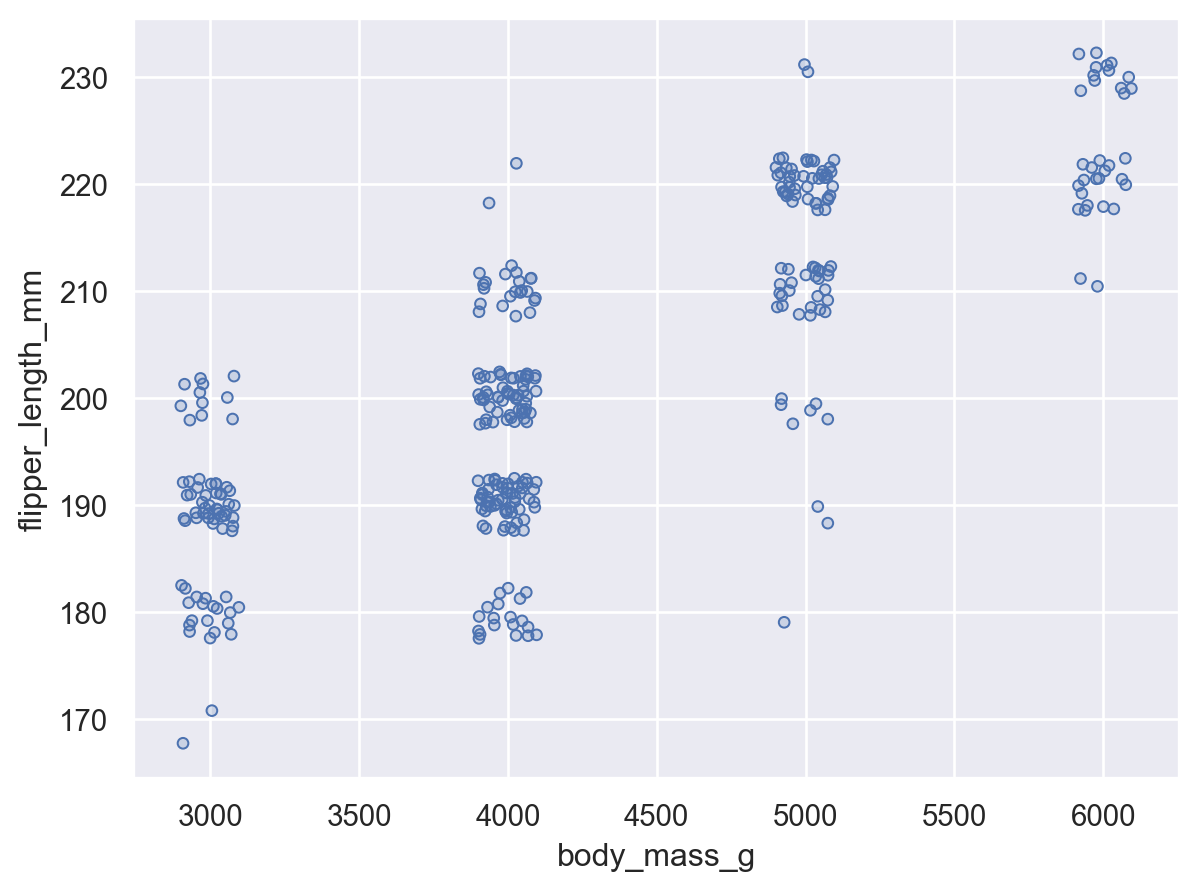# seaborn.objects.Jitter#

class seaborn.objects.Jitter(width=<default>, x=0, y=0, seed=None)#

Random displacement along one or both axes to reduce overplotting.

Parameters:
widthfloat

Magnitude of jitter, relative to mark width, along the orientation axis. If not provided, the default value will be 0 when `x` or `y` are set, otherwise there will be a small amount of jitter applied by default.

xfloat

Magnitude of jitter, in data units, along the x axis.

yfloat

Magnitude of jitter, in data units, along the y axis.

Examples

When used without any arguments, a small amount of jitter will be applied along the orientation axis:

```(
so.Plot(penguins, "species", "body_mass_g")
)
```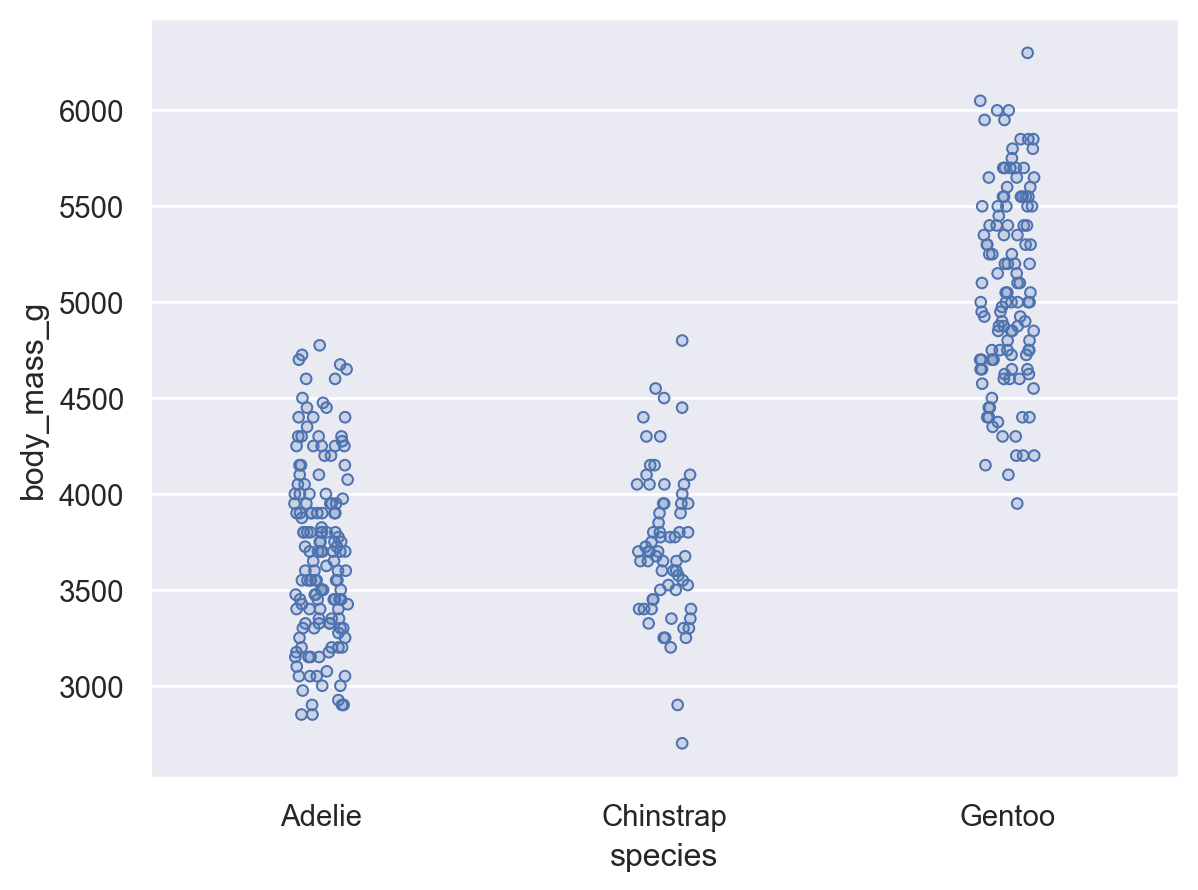The `width` parameter controls the amount of jitter relative to the spacing between the marks:

```(
so.Plot(penguins, "species", "body_mass_g")
)
```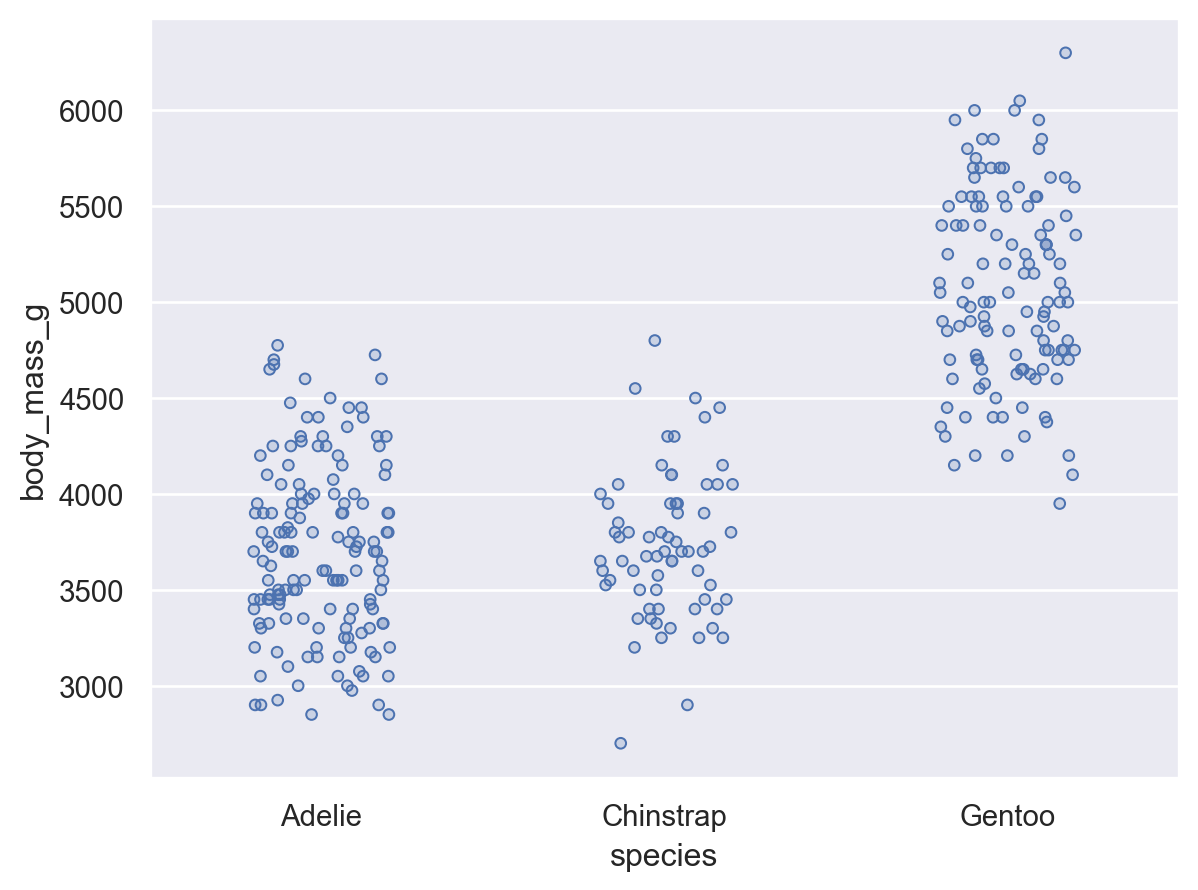The `width` parameter always applies to the orientation axis, so the direction of jitter will adapt along with the orientation:

```(
so.Plot(penguins, "body_mass_g", "species")
)
```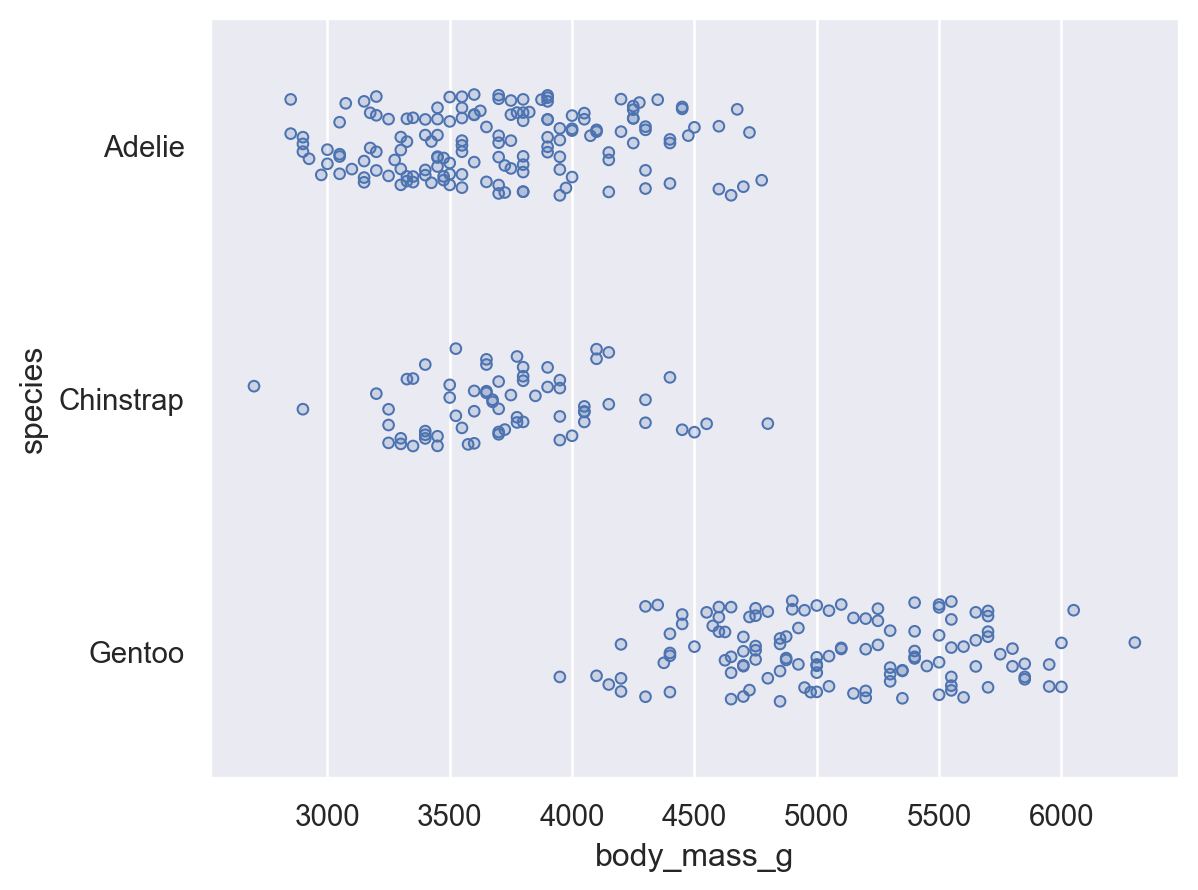Because the `width` jitter is relative, it can be used when the orientation axis is numeric without further tweaking:

```(
so.Plot(penguins["body_mass_g"].round(-3), penguins["flipper_length_mm"])
)
```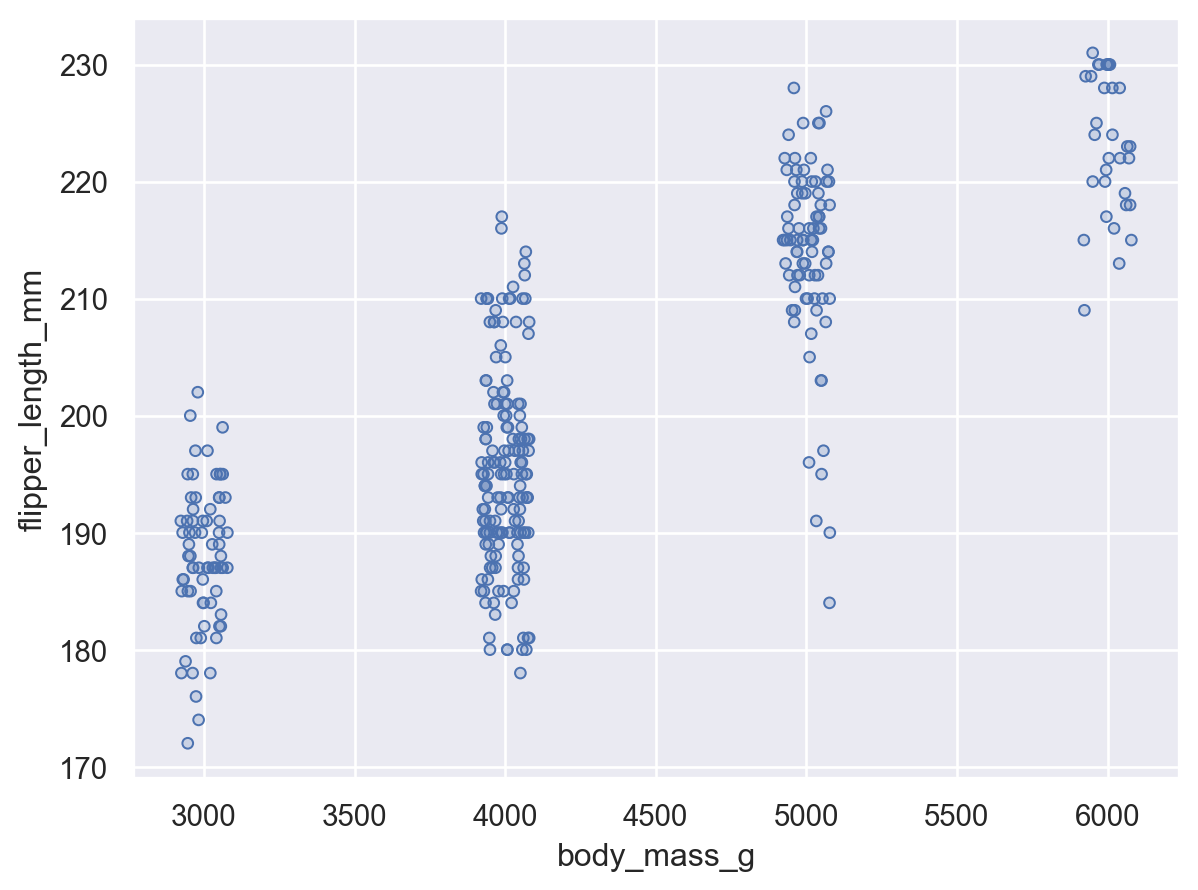In contrast to `width`, the `x` and `y` parameters always refer to specific axes and control the jitter in data units:

```(
so.Plot(penguins["body_mass_g"].round(-3), penguins["flipper_length_mm"])
)
```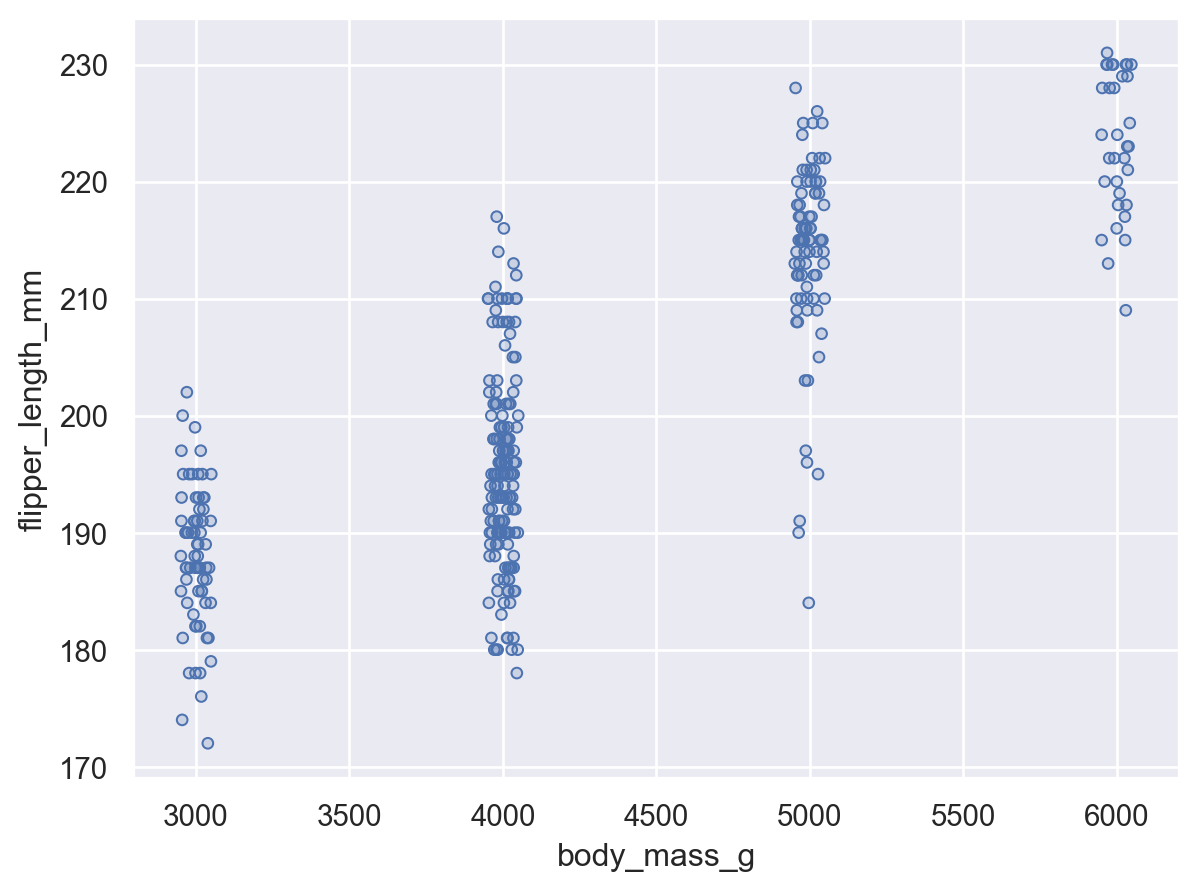Both `x` and `y` can be used in a single transform:

```(
so.Plot(
penguins["body_mass_g"].round(-3),
penguins["flipper_length_mm"].round(-1),
)